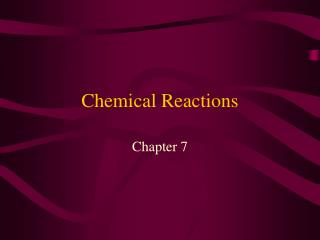DownloadDownload PresentationChemical Reactions

# Chemical Reactions

Télécharger la présentation## Chemical Reactions

- - - - - - - - - - - - - - - - - - - - - - - - - - - E N D - - - - - - - - - - - - - - - - - - - - - - - - - - -
##### Presentation Transcript

1. Chemical Reactions Chapter 7

2. Chemical Equations • A way to describe what happens in a chemical reaction. • Tells us what substances are involved with the reaction • Tells us how much of each substance is needed in a chemical reaction

3. REACTANTS The substances that go into a chemical equation. Left side of the arrow PRODUCTS The substances produced or made in a chemical reaction Right side of the arrow Reactants & Products

4. What do the numbers mean? • Coefficients : the numbers placed in front of an element or compound. Tells us how many molecules or units of that substance are present. 3 NaOH • Subscripts : the small numbers placed after and below an element or ion. Tells us how many atoms are present. CaCl2

5. More chemical equations: • Magnesium + oxygen magnesium oxide • Mg + O2 MgO • What is wrong with this equation?

6. Balancing Chemical Equations • Can only add or change coefficients NEVER subscripts. • Balance hydrogen atoms last. • Balance oxygen atoms second to last.

7. Law of Conservation of Mass • Matter can neither be created nor destroyed. • Developed by Antoine Lavoisier (1743-1794)

8. The Mole • A special unit in chemistry used to measure the number of particles in a specific amount of mass. • One mole equals exactly 6.02 x 1023 atoms. (Avogadro’s number)

9. A mole is used the same way as: A pair a ream a dozen

10. Working problems with moles • 1 mole of iron would have a mass of how many grams? • 64 grams of sulfur is equal to how many moles? • 1/2 or 0.5 moles of water has a mass of ?

11. 1. Synthesis reactions • A type of chemical reaction where two or more reactants combine to form a single substance. • General equation: • A + B AB

12. Examples of synthesis reactions: • Formation of salt from chlorine gas and solid sodium. • Magnesium oxide formation. • Rusting of metals

13. 2. Decomposition reactions • When a complex substance breaks down into two or more simpler substances. • Generic equation: • AB A + B

14. Decomposition examples • Electrolysis of water into hydrogen gas and oxygen gas. • Production of cement. • Sodium azide in safety air bags. Page 201

15. Single-Replacement reactions • When an uncombined element replaces another element in compound. • Generic equation: • A + BC AC + B • Which of the two combined elements are replaced?

16. “Like” replaces “like” • Metals replace metals and non-metals replace non-metals. • Examples: • Zinc + copper sulfate • Calcium + water • Fluorine gas + potassium bromide

17. 4. Double Replacement • Also known as ionic exchange reactions. • Generic equation: • AB + CD AD + CB • Example: • Lead nitrate + potassium iodide

18. 5. Combustion reactions • When a substance rapidly reacts with oxygen to produce light and/or heat. • “burning” • Example: • Bunsen burner: methane + oxygen • Sometimes these reactions are classified as synthesis reactions.

19. OXIDATION When a metal combines with oxygen. Rusting Any process where an element loses electrons REDUCTION When an element gains electrons during a reaction. REDOX

20. Energy changes in chemical reactions • 2 things that always change during a chemical reaction: • The properties of the reactants. • The amount of energy present. • Energy change occurs because of breaking and formation of chemical bonds.

21. Exothermic reactions • A chemical reaction that releases energy to its surroundings. • Often in the form of heat. Reaction feels warm or hot. • Examples: • Mg + HCL

22. Endothermic reactions • A chemical reaction that absorbs energy from its surroundings. • Often feel cool. • Examples: • Ice-packs

23. Exothermic Endothermic Energy diagrams

24. Reaction rates • Defined as how quickly a reaction occurs. • Collision theory = The more collisions that occur at the atomic level the faster the reaction will go

25. Factors that affect rate of reaction • Temperature • Surface Area • Stirring • Concentration • Catalysts

26. TEMPERATURE • Generally, the higher the temperature of the reactants the faster the rate of reaction.

27. SURFACE AREA • The greater the surface area, the faster the rate of reaction. • Example:

28. STIRRING • Speeds up the molecules thus increasing the number of collisions

29. CONCENTRATION • Defined as the number of particles in a given unit of volume. • Example: • 12 M HCl • 6 M HCl • 1 M HCl

30. CATALYSTS • A substance that speeds up a reaction without being used up or directly involved in the reaction.